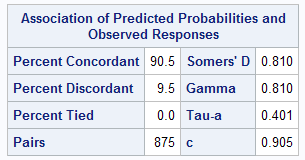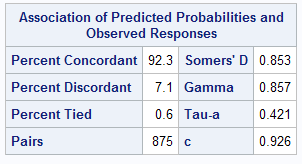# Detecting Interaction in Regression Model

This tutorial talks about the easy and effective method to detect interaction in a regression model.

What is Interaction

Interaction is defined as a combinations of variables. If the dependent variable is Y and there is an interaction between two predictors X1 and X2, it means that the relationship between X1 and Y differs depending on the value of X2.

Example -

Suppose you need to predict an employee attrition - whether an employee will leave the organisation or not (Binary - 1 / 0). Employee Attrition is dependent on various factors such as Tenure within the organization, educational qualification, last year rating (, type of job, skill type etc.

Let's build a simple predictive employee attrition model -

For demonstration, take only two independent variables - Tenure within the organization (Tenure) and Last Year Rating (Rating. Two categories - Average / Above Average). Target Variable - Attrition (1/0). The logistic regression equation looks like below -
logit(p) = Intercept + B1*(Tenure) + B2*(Rating)

Adding Interaction of Tenure and Rating

Adding interaction indicates that the effect of Tenure on the attrition is different at different values of the last year rating variable. The revised logistic regression equation will look like this:

logit(p) = Intercept + B1*(Tenure) + B2*(Rating) + B3*Tenure*Rating

Run Logistic Regression without Interaction

In SAS, you can run logistic regression with PROC LOGISTIC.
proc logistic data = mydata;
class Rating;
model Attrition = Tenure Rating;
run;Model Statistics
The c-statistics is 0.905. It is also called Area under Curve (AUC). It is an important metrics which helps to compare models.

Run Logistic Regression with Interaction
proc logistic data = mydata;
class Rating;
model Attrition = Tenure | Rating @2 / selection = stepwise slentry=0.15 slstay=0.20;
run;
To include all possible interactions, you can use '|' in the MODEL statement of PROC LOGISTIC. The @n specifies the number of predictors that can be involved in an interaction. For example, '@2' refers to 2-way interactions. @3 refers to3-way interactions. In this code, the two way interactions refers to main effects - Tenure, Rating and Interaction - Tenure * Rating

In the code, we are performing stepwise logistic regression which considers 0.15 significance level for adding a variable and 0.2 significance level for deleting a variable.Model Statistics - Model II
AUC score has increased from 0.905 to 0.926. It means it's worth adding interaction in the predictive model.

Important Points to Consider
1. Make sure you check both training and validation scores when adding interactions. It is because adding interaction may overfit the model.
2. Check AUC and Lift in top deciles while comparing models.
3. Make sure no break in rank ordering when interactions are included.
4. Adding transformed variables with Interactions make model more robust.
5. You can add more than 2-way interactions but that would be memory intensive.Share
Related Posts